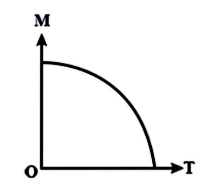Courses
Courses for Kids
Free study material
Offline Centres
MoreLast updated date: 02nd Dec 2023
Total views: 280.5k
Views today: 5.80k

# A curve between magnetic moment and temperature of magnet is ?Verified
280.5k+ views
Hint:Let us first get some idea about magnetic moment and Curie temperature. The magnetic moment is the magnetic power and orientation of a magnet or other magnetic field-producing object. The Curie temperature, also known as the Curie point, is the temperature at which some materials lose their permanent magnetic properties and are replaced by induced magnetism.

Let us first see the curve then we will jump to the explanation.Here, $M$= Magnetic Moment and $T$= Curie temperature.

As seen in the diagram, the magnetic moment varies with temperature. Curie's temperature is the temperature at which a magnetic substance loses its magnetism. As a result, the temperature of Curie is found at the point where the curve intersects the $T$ axis.

The magnetic moment is a property that describes how strong a magnet can pull on the electric field lines. The position of the magnetic moment can be determined using the right hand thumb law. The Curie temperature is the point at which the properties of magnetic materials change dramatically.

At zero temperature, magnetic moments in opposite directions have zero net magnetic moment. When the temperature of a substance rises, the particles or atoms begin to vibrate more. The magnetism increases as the temperature rises. If the vibrations continue to rise, the temperature will drop to zero. Just above the Curie temperature does this happen. The graph is a rising curve before the Curie temperature. The graph begins to decrease as soon as the Curie temperature is obtained.

Note:So from the above discussion we can say that the magnetic moment is a vector quantity that measures an object's proclivity to interact with a magnetic field and the magnetism of a magnet decreases as temperature rises, and it is essentially zero above the Curie temperature graph.